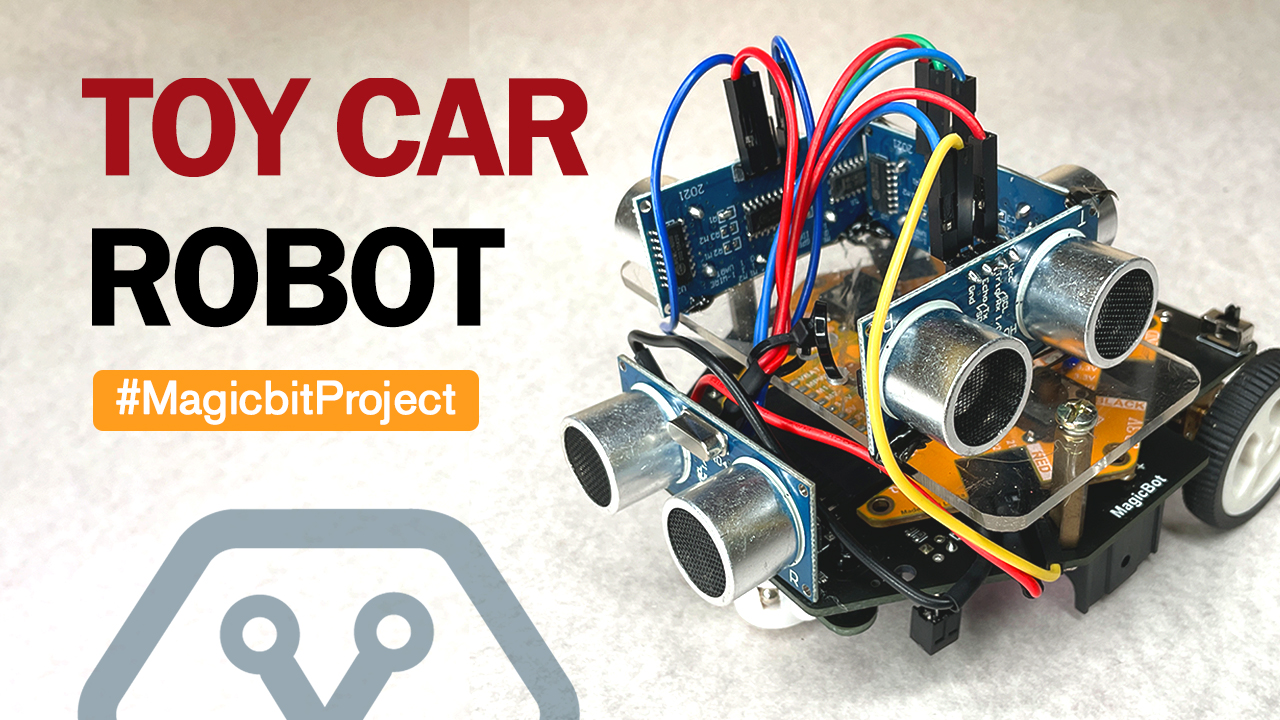### Toy Car

#### Components Required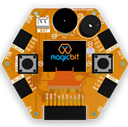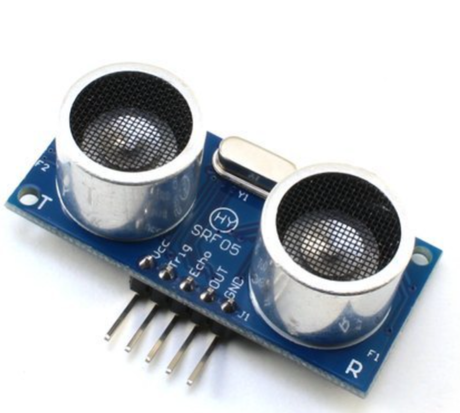#### Introduction

In this project, we will make a toy robot car. Whenever someone or something gets too close to it, it will move away. The closer you are to the car, it will try to move away faster from you. This might be a good toy for your pet dog/cat.

#### What You Need

• Magicbit
• Magicbot
• Three Ultrasonic sensors.
• Batteries#### Don't have a Magicbot?

If you do not have a magicbot, do not worry. You can use four Ultrasonic sensors. Also you will have to make your own custom robot that can carry all the four ultrasonic sensors, the board and the battery.

#### Circuit and pin connections

First, let’s figure out the pin connections for the ultrasonic sensors. You can connect the Trigger and Echo pin together as shown below in the diagram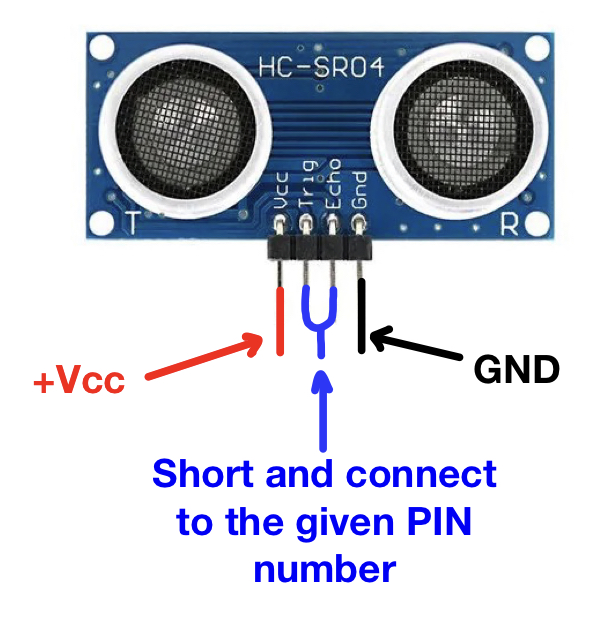It is easier if you make a mount and place the sensors on the mount and then position the mount on the magicbot. You can make a mount as shown below for an example.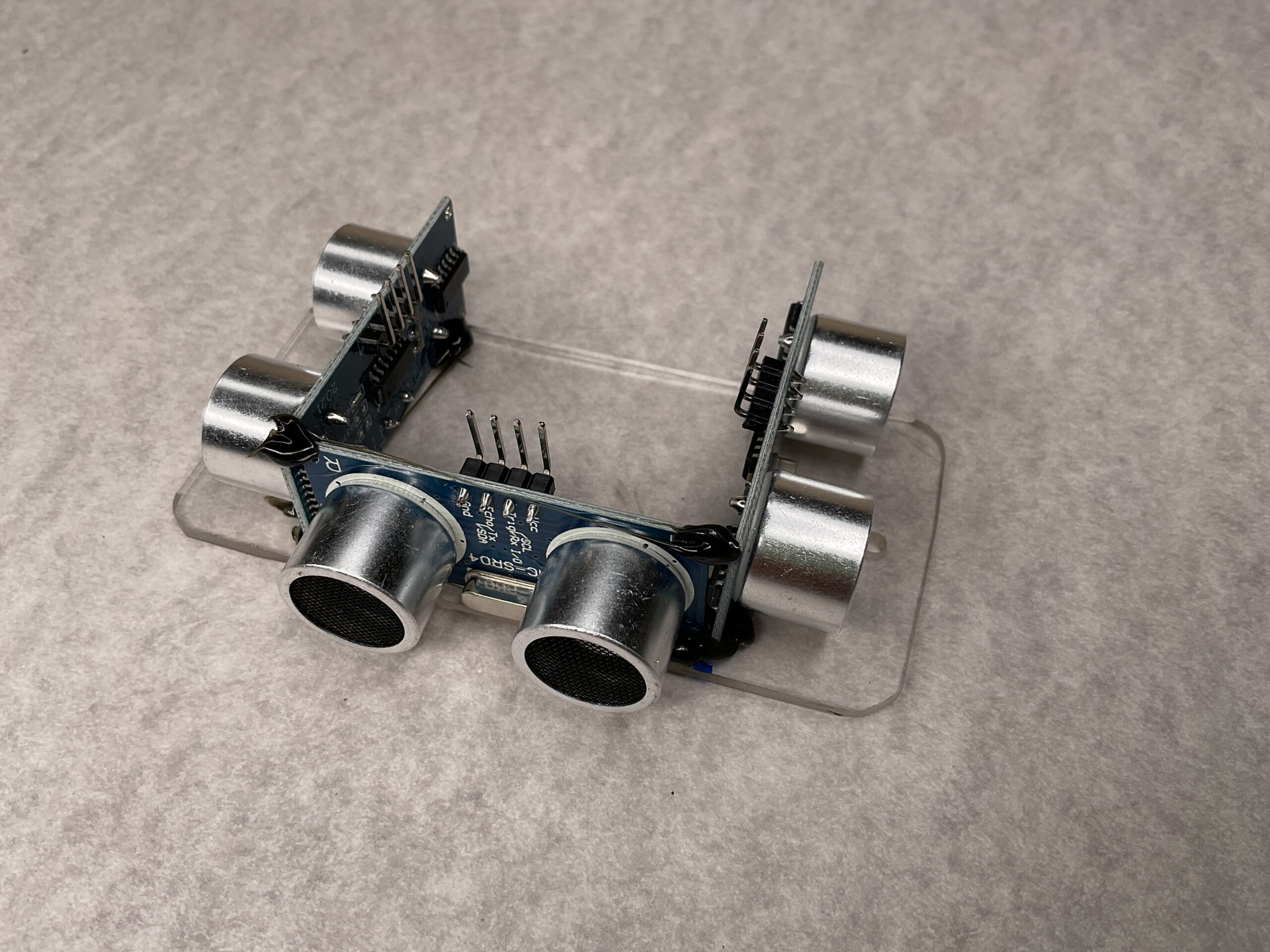After mounting the sensors, our toy Robot car looks like this!Remember that you have to position them on the magicbot in four directions as shown below (F-Forward, B-Backword, etc)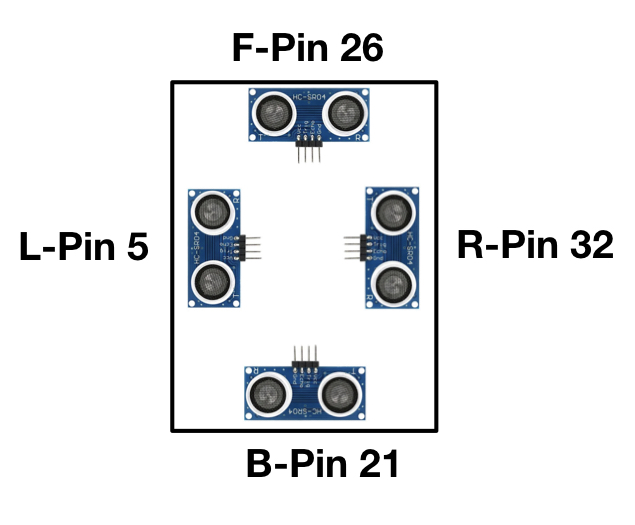Connect the pins to the relevant ports on Magicbit with jumper cables as shown in the above image. After everything is done correctly, our toy car looks like this.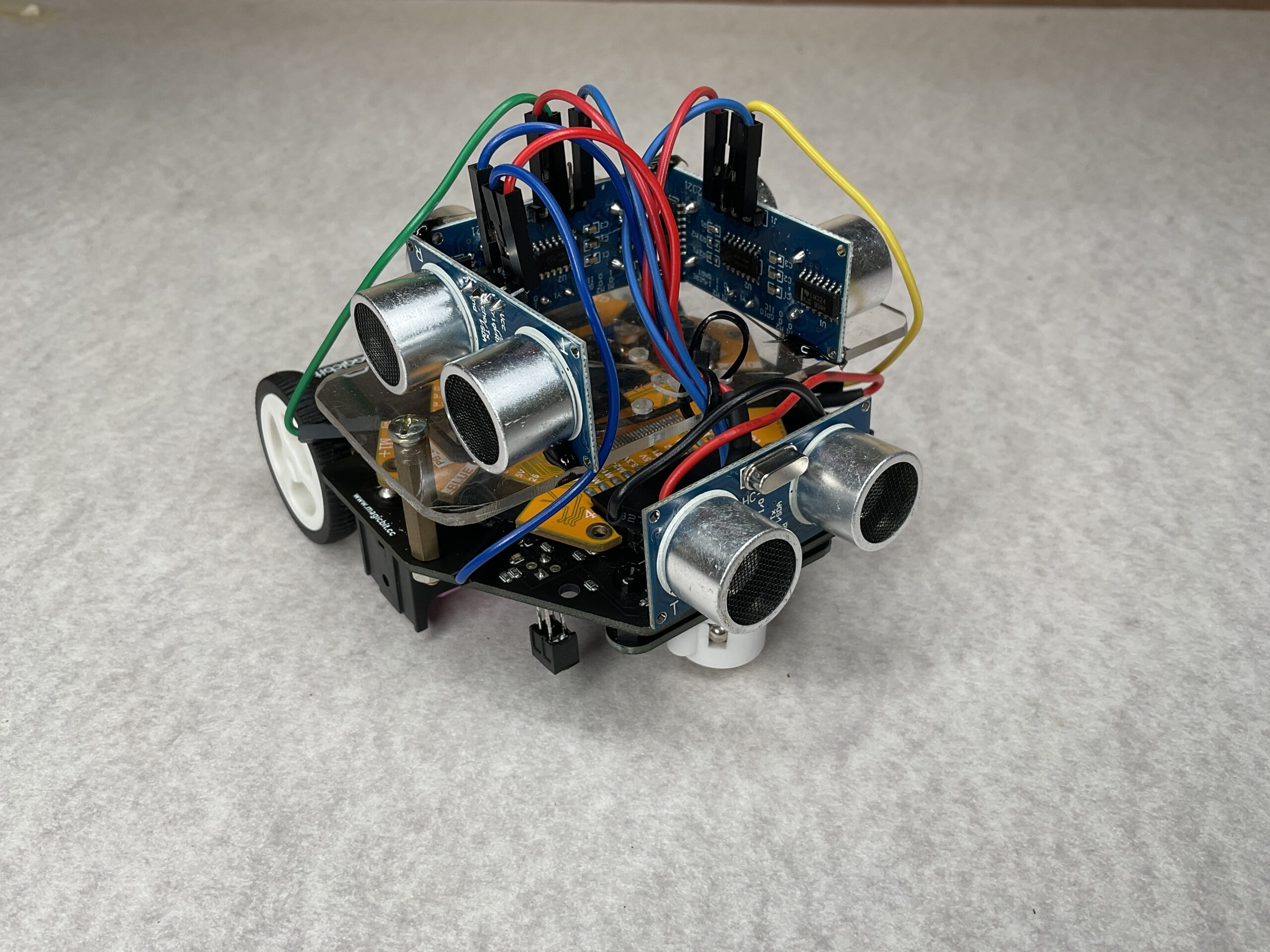• Left motor is connected to pins 16 and 17.
• Right motor is connected to pins 18 and 27

Note that magicbit has inbuilt motor drivers.

#### Code

Upload the given code to the magicbit before installing it on magicbot.

```#include <ESP32Servo.h>
#include <NewPing.h>
#define T_F    26  // Trigger pin
#define E_F    26  // Echo pin

#define T_B    21
#define E_B    21

#define T_L    5
#define E_L    5

#define T_R    32
#define E_R    32

#define MAX_DISTANCE 200

NewPing sonar_F(T_F, E_F, MAX_DISTANCE);
NewPing sonar_B(T_B, E_B, MAX_DISTANCE);
NewPing sonar_L(T_L, E_L, MAX_DISTANCE);
NewPing sonar_R(T_R, E_R, MAX_DISTANCE);

int limit = 20; // Minimum distance that the car starts to move.

int M1A = 17; //motor drive input pins
int M1B = 16;
int M2A = 27;
int M2B = 18;
int F;
int B;
int L;
int R;

void setup() {
// put your setup code here, to run once:
//Motor inputs
pinMode(M1A, OUTPUT);
pinMode(M1B, OUTPUT);
pinMode(M2A, OUTPUT);
pinMode(M2B, OUTPUT);

Serial.begin(115200);

}

void loop() {
// Get data from sonars
F = sonar_F.ping_cm();
delay(10);
B = sonar_B.ping_cm();
delay(10);
L = sonar_L.ping_cm();
delay(10);
R = sonar_R.ping_cm();
delay(10);

/*
Serial.print(F);
Serial.print(" ");
Serial.print(B);
Serial.print(" ");
Serial.print(L);
Serial.print(" ");
Serial.print(R);
Serial.println(" ");
*/

// Method
if (F<limit) {
reverse(Speed(F));
}

if (B<limit) {
forward(Speed(B));
}
if (L<limit) {
turn_L(Speed(L));
}
if (R<limit) {
turn_R(Speed(R));
}

if ((F>limit)||(B>limit)||(L>limit)||(R>limit)) {
analogWrite(M1A, 0);
analogWrite(M1B, 0);
analogWrite(M2A, 0);
analogWrite(M2B, 0);
delay(200);
}
}

void forward(int x) {  //x is the speed
analogWrite(M1A, x);
analogWrite(M1B, 0);
analogWrite(M2A, x);
analogWrite(M2B, 0);
delay(200);
}

void reverse(int x) {  //x is the speed
analogWrite(M1A, 0);
analogWrite(M1B, x);
analogWrite(M2A, 0);
analogWrite(M2B, x);
delay(200);
}

void turn_L(int x) {  //x is the speed
analogWrite(M1A, 0);
analogWrite(M1B, x);
analogWrite(M2A, x);
analogWrite(M2B, 0);
delay(200);
}

void turn_R(int x) {  //x is the speed
analogWrite(M1A, x);
analogWrite(M1B, 0);
analogWrite(M2A, 0);
analogWrite(M2B, x);
delay(200);
}

int Speed(int distance) {
return map(distance,40,10,0,255);
}

```

#### Troubleshooting

• If you want to change the response distance, change the limit variable in the program to the minimum distance that you want your car to start motion.
• If you are not using Magicbot, you might have to figure out the motor rotation direction on your own. Try changing motor pins(M1A with M1B and M2A with M2B) Try a few combinations to figure out their rotation directions(Refer to the functions in the code).